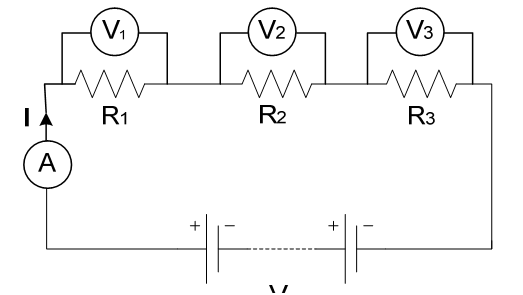## Explain series circuit, Electrical Engineering

Assignment Help:

Series circuit

Total resistance, RT = R1 + R2 + R3

The current I is the same in all parts of the circuit , I = I1 = I2 = I3

The voltage drop at each resistor, V1, V2 and V3 are different depends on the value of the resistor.

The sum of the voltages V1, V2 and V3 is equal to the total applied voltage, V = V1 = V2 = V3#### Explain atomic structure and energy band diagram of silicon, Explain Atomic...

Explain Atomic structure and Energy Band Diagram of Silicon. Silicon : It is other most commonly used semiconductor. Atomic number of it is 14. The no. of electrons in first,

#### What is programmable interrupt controller, What is programmable interrupt c...

What is programmable interrupt controller? The Programmable interrupt controller is used when several I/O devices transfer datausing interrupt and they are connected to the sam

#### Circuit theory, Maximum power transfer theorem

Maximum power transfer theorem

#### Conductometry experiment, why conductance is not zero at end pont? reply as...

why conductance is not zero at end pont? reply as soon as possible.

#### Digital control system, block diagram of digital control system and explain...

block diagram of digital control system and explain each block

#### Explain how switching systems are classified, Question 1 Explain how switc...

Question 1 Explain how switching systems are classified Question 2 Explain the function of pulse dialing in rotary dial telephone Question 3 Explain the functioning of crossba

#### Change management in power distribution, Change Management in Power Distrib...

Change Management in Power Distribution: Organisational change might be described as an organisation-wide effort to augment the effectiveness of an organisation through str

#### #title.electricity, what does electricity means?

what does electricity means?

#### Magnets, B-H curve of SM2CO17

B-H curve of SM2CO17

#### What is a clipper circuit, Q. What is a clipper circuit? Clipping circu...

Q. What is a clipper circuit? Clipping circuits are linear wave shaping circuits. The main function of 'clipper' is that it clips off a part of input waveform i.e., it cut off